# Oscar awards: good actor versus good actressI am not a big fan of those ceremonies, where some actors pretend that they are extremely happy to be there, and then some win a trophy, some don’t, and those who win start to cry, and those who did not get a trophy try to pretend that they are not affected, etc. The other reason is that, since I have several kids, I do not go to see the movies that often (I mean apart from Shrek, Toy Story… Harry Potter is probably the only movie I’ve seen with real actors – or at least human actors).

But I remember being surprised when I looked at the nominees in newspapers,Actresses are beautiful and look young, while actors are more experienced. So I have try to see how old were those who win an Oscar, as best actor (here) or best supporting actor (there), and best actress (here) and best supporting actress (there).

```OSCAR=read.table("http://freakonometrics.blog.free.fr/public/data/OSCAR.csv",
actor=OSCAR[,1]
suppactor=OSCAR[,2]
actress=OSCAR[,3]
suppactress=OSCAR[,4]
actor=actor[is.na(actor)==FALSE]
actor=actor[actor>0]
actress=actress[is.na(actress)==FALSE]
actress=actress[actress>0]
suppactor=suppactor[is.na(suppactor)==FALSE]
suppactor=suppactor[suppactor>0]
suppactress=suppactress[is.na(suppactress)==FALSE]
suppactress=suppactress[suppactress>0]

boxplot(actor,suppactor,actress,suppactress,col=c("blue","blue","red","red"),
names=c("actor","supp. actor","actress","supp. actress"))```On average, a best actress is 36 years old, while a best actor is 44 years old.  Which is quite a difference… Perhaps because it takes more time to an actor to be a good one ? Assuming that they start acting at 18, it takes 18 more years for an actress to be recognized as a good one (here the best one), and 26 for an actor. Or perhaps it is simply because leading actresses have to look young…
The oldest actor who won an Oscar was Henry Fonda (at the age of 76) and the oldest actress was Jessica Tendy (nearing 81). Tatum O’Neal became the youngest person to win the best suppo
rting actress award
at the age of 10 (she was 8 when she was acting). The youngest best actress was Marlee Matlin, 21. The distribution was be seen below, with actors in blue, and actresses in red, best supporting actors in dotted lines, and best actors in plain lines,

```plot(density(actor),xlim=c(10,80),axes=FALSE,
col="blue",names="",ylab="",xlab="",ylim=c(0,.051))
lines(density(suppactor),col="blue",lty=2)
lines(density(actress),col="red")
lines(density(suppactress),col="red",lty=2)
axis(1)```Note that the age of supporting actors is older that leading ones. E.g. the average age for supporting actors winning an Oscar is 50, while it is  44 for actors. Similarly, it is 40 for supporting actresses, and 36 for actresses.

```> mean(suppactor)
 50.23762

> mean(actor)
 44.29982

> mean(suppactress)
 40.55766

> mean(actress)
 36.39733```

Here, I have to admit that I was surprised. I always thought that being a supporting actor was a first step before being a leading one. So winners of supporting awards should have been younger that winners of leading ones. But this is not the case.

And the dynamic here is rather stable, with actors,and actresses,except that the age difference between supporting roles and leading roles have increased in the 80’s for actors, while it decreased in the 80’s for actresses.

# Open data might be a false good opportunity…

I am always surprised to see many people on Twitter tweeting about #opendata, e.g. @data4all, @usdatagov, @datapublicatwit, @ProPublica or @open3 among so many others… Initially, I was also very enthousiastic, but I have to admit thatopen data are rarely raw data. Which is what I am usually looking for, as a statistician…
Consider the following example: I was wondering (Valentine’s day is approaching)when will a man born in 1975 (say) get married – if he ever gets married ?More technically, I was looking for a distribution of the age of first marriage (given the year of birth), including the proportion of men that will never get married, for that specific cohort.The only data I found on the internet is the following, on statistics.gov.uk/Note that we can also focus on women (e.g. here). Is it possible to use that opendata to get an estimation of the distribution of first marriage for some specific cohort ? (and to answer the question I asked). Here, we have two dimensions: on line, the year (of the marriage), and on column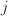, the age of the man when he gets married. Assume that those were rawdata, i.e. that we have the number of marriages of men of ageduring the year.

We are interested at a longitudinal lecture of the table, i.e. consider some man born year, we want to estimate (or predict) the age he will get married, if he gets married. With raw data, we can do it… The first step is to build up triangles (to have a cohort vs. age lecture of the data), and then to consider a model, e.g.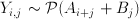where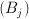is a year effect, and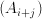is a cohort effect.

```base=read.table("http://freakonometrics.free.fr/mariage-age-uk.csv",
m=base[1:16,]
m=m[,3:10]
m=as.matrix(m)
triangle=matrix(NA,nrow(m),ncol(m))
n=ncol(m)
for(i in 1:16){
triangle[i,]=diag(m[i-1+(1:n),])
}
triangle[nrow(m),1]=m[nrow(m),1]

triangle
[,1] [,2] [,3] [,4] [,5] [,6] [,7] [,8]
[1,]   12  104  222  247  198  132   51   34
[2,]    8   89  228  257  202  102   75   49
[3,]    4   80  209  247  168  129   92   50
[4,]    4   73  196  236  181  140   88   45
[5,]    3   78  242  206  161  114   68   47
[6,]   11  150  223  199  157  105   73   39
[7,]   12  117  194  183  136   96   61   36
[8,]   11  118  202  175  122   92   62   40
[9,]   15  147  218  162  127   98   72   48
[10,]   20  185  204  171  138  112   82   NA
[11,]   31  197  240  209  172  138   NA   NA
[12,]   34  196  233  202  169   NA   NA   NA
[13,]   35  166  210  199   NA   NA   NA   NA
[14,]   26  139  210   NA   NA   NA   NA   NA
[15,]   18  104   NA   NA   NA   NA   NA   NA
[16,]   10   NA   NA   NA   NA   NA   NA   NA

Y=as.vector(triangle)
YEARS=seq(1918,1993,by=5)
AGES=seq(22,57,by=5)
X1=rep(YEARS,length(AGES))
X2=rep(AGES,each=length(YEARS))
reg=glm(Y~as.factor(X1)+as.factor(X2),family="poisson")
summary(reg)

Call:
glm(formula = Y ~ as.factor(X1) + as.factor(X2), family = "poisson")

Deviance Residuals:
Min       1Q   Median       3Q      Max
-5.4502  -1.1611  -0.0603   1.0471   4.6214

Coefficients:
Estimate Std. Error z value Pr(>|z|)
(Intercept)        2.8300461  0.0712160  39.739  < 2e-16 ***
as.factor(X1)1923  0.0099503  0.0446105   0.223 0.823497
as.factor(X1)1928 -0.0212236  0.0449605  -0.472 0.636891
as.factor(X1)1933 -0.0377019  0.0451489  -0.835 0.403686
as.factor(X1)1938 -0.0844692  0.0456962  -1.848 0.064531 .
as.factor(X1)1943 -0.0439519  0.0452209  -0.972 0.331082
as.factor(X1)1948 -0.1803236  0.0468786  -3.847 0.000120 ***
as.factor(X1)1953 -0.1960149  0.0470802  -4.163 3.14e-05 ***
as.factor(X1)1958 -0.1199103  0.0461237  -2.600 0.009329 **
as.factor(X1)1963 -0.0446620  0.0458508  -0.974 0.330020
as.factor(X1)1968  0.1192561  0.0450437   2.648 0.008107 **
as.factor(X1)1973  0.0985671  0.0472460   2.086 0.036956 *
as.factor(X1)1978  0.0356199  0.0520094   0.685 0.493423
as.factor(X1)1983  0.0004365  0.0617191   0.007 0.994357
as.factor(X1)1988 -0.2191428  0.0981189  -2.233 0.025520 *
as.factor(X1)1993 -0.5274610  0.3241477  -1.627 0.103689
as.factor(X2)27    2.0748202  0.0679193  30.548  < 2e-16 ***
as.factor(X2)32    2.5768802  0.0667480  38.606  < 2e-16 ***
as.factor(X2)37    2.5350787  0.0671736  37.739  < 2e-16 ***
as.factor(X2)42    2.2883203  0.0683441  33.482  < 2e-16 ***
as.factor(X2)47    1.9601540  0.0704276  27.832  < 2e-16 ***
as.factor(X2)52    1.5216903  0.0745623  20.408  < 2e-16 ***
as.factor(X2)57    1.0060665  0.0822708  12.229  < 2e-16 ***
---
Signif. codes:  0 ‘***’ 0.001 ‘**’ 0.01 ‘*’ 0.05 ‘.’ 0.1 ‘ ’ 1

(Dispersion parameter for poisson family taken to be 1)

Null deviance: 5299.30  on 99  degrees of freedom
Residual deviance:  375.53  on 77  degrees of freedom
(28 observations deleted due to missingness)
AIC: 1052.1

Number of Fisher Scoring iterations: 5```

Here, we have been able to derive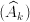and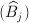, where now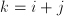denotes the cohort.
We can now predict the number of marriages per year, and per cohortHere, given the cohort, the shape of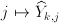is the following

```Yp=predict(reg,type="response")
tYp=matrix(Yp,nrow(m),ncol(m))
tYp[16,]
tYp[16,]
  10.00000 222.94525 209.32773 159.87855 115.06971  42.59102
  18.70168 148.92360```The errors (Pearson error) look like that
`Ep=residuals(reg,type="pearson")`(where the darker the blue, the smaller the residuals, and the darker the red, the higher the residuals). Obviously, we are missing something here, like a diagonal effect. But this is not the main problem here…I guess that study here is not valid. The problem is that we deal with open data, and numbers of marriages are not given here: what is given is a he proportion of marriage of men of ageduring the year, with a yearly normalization. There is a constraint on lines, i.e. we observe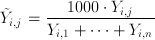so that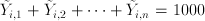This is mentioned in the titleIt is still possible to consider a Poisson regression on the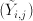, but unfortunately, I do not think any interpretation is valid (unless demography did not change last century). For instance, the following sum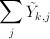looks like that

```apply(tYp,1,sum)
 919.948 838.762 846.301 816.552 943.559 930.280 857.871 896.113
 905.086 948.087 895.862 853.738 826.003 816.192 813.974 927.437```

i.e. if we look at the graphBut I do not think we can interpret that sum as the probability (if we divide by 1,000) that a man in that cohort gets married…. And more basically, I cannot do anything with that dataset…So open data might be interesting. The problem is that most of the time, the data are somehow normalized (or aggregated). And then, it becomes difficult to use them…

So I will have to work further to be able to write something (mathematically valid) on marriage strategy before Valentine’s day…. to be continued.